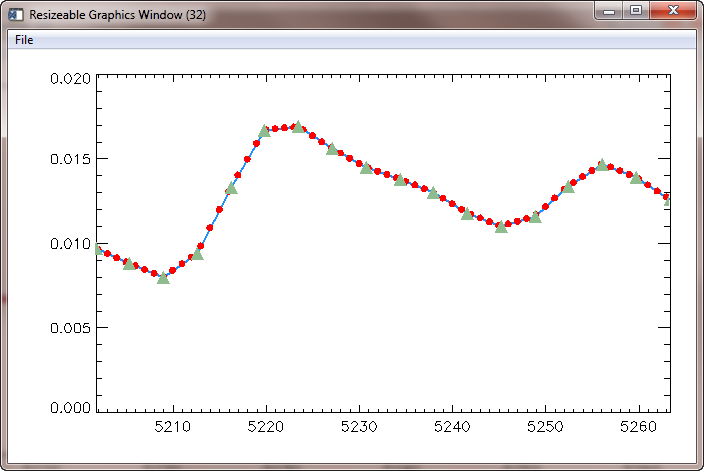Interpolating Flux to Regular Wavelengths

QUESTION: I have my data in two columns. The first column is the wavelength in angstroms and the second column is the flux. The data looks like this.

wavelength          flux
5201.720215        0.0097
5205.345215        0.0088
5208.970703        0.0080
5212.596191        0.0094
5216.221680        0.0133
5219.846680        0.0167
5223.472168        0.0169
5227.097656        0.0156
5230.722656        0.0145
5234.348145        0.0138
5237.973633        0.0130
5241.599121        0.0118
5245.224121        0.0110
5248.849609        0.0116
5252.475098        0.0134
5256.100586        0.0147
5259.725586        0.0139
5263.351074        0.0126

I want to create a new data set from this data that is sampled or re-binned on 1 angstrom intervals, with the flux linearly interpolated from the data I have collected. I am confused about which routines in IDL I should use (Rebin, Congrid, Interpolate, etc.) to do this. Can you show me how this can be done?Since your data is already ordered in a monotonically increasing way and is the same data type, we can just create a two-dimensional array of the data.

data = [ [5201.720215d, 0.0097],\$
[5205.345215d, 0.0088],\$
[5208.970703d, 0.0080],\$
[5212.596191d, 0.0094],\$
[5216.221680d, 0.0133],\$
[5219.846680d, 0.0167],\$
[5223.472168d, 0.0169],\$
[5227.097656d, 0.0156],\$
[5230.722656d, 0.0145],\$
[5234.348145d, 0.0138],\$
[5237.973633d, 0.0130],\$
[5241.599121d, 0.0118],\$
[5245.224121d, 0.0110],\$
[5248.849609d, 0.0116],\$
[5252.475098d, 0.0134],\$
[5256.100586d, 0.0147],\$
[5259.725586d, 0.0139],\$
[5263.351074d, 0.0126] ]

Next, we need to create a vector representing the wavelengths that we want to include in the new data set. In this case, we need a vector going from 5201 to 5264 angstroms.

start_wl = Floor(Min(data[0,*]))
end_wl = Ceil(Max(data[0,*]))
wl_vector = cgScaleVector(Findgen(end_wl-start_wl+1), start_wl, end_wl)

Next, we simply linearly interpolate the flux at the locations given by this new wavelength vector. We use the Interpol command in IDL to do this.

interp_flux = Interpol(data[1,*], data[0,*], wl_vector)

We can plot the data to see how we have done. The original data is drawn with a blue line. The newly interpolated flux data is overplotted in red symbols. The original data points are overplotted in green symbols.

cgPlot, data[0,*], data[1,*], Color='dodger blue', XStyle=1, Thick=2, /Window
cgPlot, wl_vector, interp_flux, Color='red', PSym=16, /Overplot, /Add
cgPlot, data[0,*], data[1,*], SymColor='light sea green', PSym=17, SymSize=2, /Overplot, /Add

You see the results in the figure below.The original and interpolated flux values.